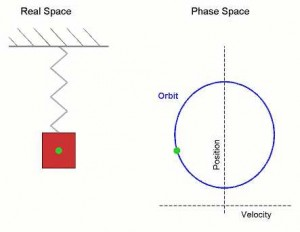# What is meant by Simple Harmonic Motion?

If the periodic and oscillatory motion of a particle is such that, its acceleration is directly proportional to the displacement from its equilibrium position of rest and is always directed towards the equilibrium position, then the motion is known as simple harmonic motion or Oscillation.

The motion of any free particle executing both periodic and oscillatory is simple harmonic.Fig.- Simple harmonic motion is shown both in real space and phase space.

Characteristics of simple harmonic or oscillation:

• The motion is linear.
• The motion is periodic.
• It is oscillatory.
• The acceleration of the oscillatory particles is directly proportional to the displacement about its equilibrium position and is directed opposite to the displacement.
• The acceleration is always directed towards the equilibrium position.
• Simple harmonic motion can be expressed as sine or cosine function.